1597696980

# What does dimensionality reduction do, really?

Picture this: A student is in art class and their teacher asks them to draw a cube on a sheet of paper. Now, as an astute participant in reality, the student realizes that they cannot draw a 3 Dimensional Object on a 2 Dimensional surface. So, with their brilliant critical thinking skills, they attempt to draw one face of the cube on your paper by connecting 4 points.incorrect projection of cube onto 2d plane || author image

This is a good attempt, but the student realizes that they have not preserved the features of the cube, as they only have one side drawn on their piece of paper. They have only drawn 4 points on their paper, while there are 8 vertices on the cube. They continue by drawing another shape:accurate projection of cube onto 2d plane || author image

They show this result to their art teacher, and the teacher is pleased with the result. The student has reduced the dimension of the cube, while still preserving characteristics that make the shape identifiable. How does this relate to t-SNE you might ask? Well, this example is the driving principle of this algorithm.

#technology #programming #machine-learning #deep learning

## Buddha Community1597741140

## Dimensionality Reduction

It is easy for us to visualize two or three dimensional data, but once it goes beyond three dimensions, it becomes much harder to see what high dimensional data looks like.

Today we are often in a situation that we need to analyze and find patterns on datasets with thousands or even millions of dimensions, which makes visualization a bit of a challenge. However, a tool that can definitely help us better understand the data is dimensionality reduction.

In this post, I will discuss t-SNE, a popular non-linear dimensionality reduction technique and how to implement it in Python using sklearn. The dataset I have chosen here is the popular MNIST dataset.

## Overview

T-Distributed Stochastic Neighbor Embedding, or t-SNE, is a machine learning algorithm and it is often used to embedding high dimensional data in a low dimensional space .

In simple terms, the approach of t-SNE can be broken down into two steps. The first step is to represent the high dimensional data by constructing a probability distribution P, where the probability of similar points being picked is high, whereas the probability of dissimilar points being picked is low. The second step is to create a low dimensional space with another probability distribution Q that preserves the property of P as close as possible.

In step 1, we compute the similarity between two data points using a conditional probability p. For example, the conditional probability of j given i represents that x_j would be picked by x_i as its neighbor assuming neighbors are picked in proportion to their probability density under a Gaussian distribution centered at _x_i _. In step 2, we let _y_i _and y_j to be the low dimensional counterparts of x_i and _x_j, _respectively. Then we consider q to be a similar conditional probability for y_j being picked by y_i and we employ a **student t-distribution **in the low dimension map. The locations of the low dimensional data points are determined by minimizing the Kullback–Leibler divergence of probability distribution P from Q.

#data-visualization #sklearn #dimensionality-reduction #data-science #machine-learning #data analysis1602817200

## Autoencoders for Dimensionality Reduction

In the previous post, we explained how we can reduce the dimensions by applying PCA and t-SNE and how we can apply Non-Negative Matrix Factorization for the same scope. In this post, we will provide a concrete example of how we can apply Autoeconders for Dimensionality Reduction. We will work with Python and TensorFlow 2.x.

## Autoencoders on MNIST Dataset

We will use the MNIST dataset of TensorFlow, where the images are 28 x 28 dimensions, in other words, if we flatten the dimensions, we are dealing with 784 dimensions. Our goal is to reduce the dimensions, from 784 to 2, by including as much information as possible.

Let’s get our hands dirty!

``````from tensorflow.keras.models import Sequential
from tensorflow.keras.layers import Dense,Flatten,Reshape
from tensorflow.keras.optimizers import SGD
from tensorflow.keras.datasets import mnist
(X_train, y_train), (X_test, y_test) = mnist.load_data()
X_train = X_train/255.0 X_test = X_test/255.0
#### Encoder
encoder = Sequential()
#### Decoder
decoder = Sequential()
#### Autoencoder
autoencoder = Sequential([encoder,decoder]) autoencoder.compile(loss="mse") autoencoder.fit(X_train,X_train,epochs=50)
encoded_2dim = encoder.predict(X_train)
## The 2D
AE = pd.DataFrame(encoded_2dim, columns = ['X1', 'X2'])
AE['target'] = y_train
sns.lmplot(x='X1', y='X2', data=AE, hue='target', fit_reg=False, size=10)
``````

#autoencoder #dimensionality-reduction #data-science #data-visualization #tensorflow1601560800

## Autoencoders for Dimensionality Reduction

In the previous post, we explained how we can reduce the dimensions by applying PCA and t-SNE and how we can apply Non-Negative Matrix Factorization for the same scope. In this post, we will provide a concrete example of how we can apply Autoeconders for Dimensionality Reduction. We will work with Python and TensorFlow 2.x.

## Autoencoders on MNIST Dataset

We will use the MNIST dataset of tensorflow, where the images are 28 x 28 dimensions, in other words, if we flatten the dimensions, we are dealing with 784 dimensions. Our goal is to reduce the dimensions, from 784 to 2, by including as much information as possible.

Let’s get our hands dirty!

#autoencoder #dimensionality-reduction #data-science #data-visualization #tensorflow1593271874

## Dimensionality Reduction in Python

This is a memo to share what I have learnt in Dimensionality Reduction in Python, capturing the learning objectives as well as my personal notes. The course is taught by Jerone Boeye, and it includes 4 chapters:

Chapter 1. Exploring high dimensional data

Chapter 2. Feature selection I, selecting for feature information

Chapter 3. Feature selection II, selecting for model accuracy

Chapter 4. Feature extractionPhoto by Aditya Chinchure on Unsplash

High-dimensional datasets can be overwhelming and leave you not knowing where to start. Typically, you’d visually explore a new dataset first, but when you have too many dimensions the classical approaches will seem insufficient. Fortunately, there are visualization techniques designed specifically for high dimensional data and you’ll be introduced to these in this course.

After exploring the data, you’ll often find that many features hold little information because they don’t show any variance or because they are duplicates of other features. You’ll learn how to detect these features and drop them from the dataset so that you can focus on the informative ones. In a next step, you might want to build a model on these features, and it may turn out that some don’t have any effect on the thing you’re trying to predict. You’ll learn how to detect and drop these irrelevant features too, in order to reduce dimensionality and thus complexity.

Finally, you’ll learn how feature extraction techniques can reduce dimensionality for you through the calculation of uncorrelated principal components.

## Chapter 1. Exploring high dimensional data

You’ll be introduced to the concept of dimensionality reduction and will learn when an why this is important. You’ll learn the difference between feature selection and feature extraction and will apply both techniques for data exploration. The chapter ends with a lesson on t-SNE, a powerful feature extraction technique that will allow you to visualize a high-dimensional dataset.

## Introduction

Dataset with more than 10 columns are considered high dimensional data.

## Finding the number of dimensions in a dataset

A larger sample of the Pokemon dataset has been loaded for you as the Pandas dataframe `pokemon_df`.

How many dimensions, or columns are in this dataset?

Answer: 7 dimensions, each Pokemon is described by 7 features.

``````In : pokemon_df.shape
Out: (160, 7)
``````

## Removing features without variance

A sample of the Pokemon dataset has been loaded as `pokemon_df`. To get an idea of which features have little variance you should use the IPython Shell to calculate summary statistics on this sample. Then adjust the code to create a smaller, easier to understand, dataset.

For the number_cols, Generation column has ‘1’ in all 160 rows.

``````In : pokemon_df.describe()
Out:
HP     Attack     Defense  Generation
count  160.00000  160.00000  160.000000       160.0
mean    64.61250   74.98125   70.175000         1.0
std     27.92127   29.18009   28.883533         0.0
min     10.00000    5.00000    5.000000         1.0
25%     45.00000   52.00000   50.000000         1.0
50%     60.00000   71.00000   65.000000         1.0
75%     80.00000   95.00000   85.000000         1.0
max    250.00000  155.00000  180.000000         1.0

# Remove the feature without variance from this list
number_cols = ['HP', 'Attack', 'Defense']
``````

For the non_number_cols, Legendary column has ‘False’ in all 160 rows.

``````In : pokemon_df[['Name', 'Type', 'Legendary']].describe()
Out:
Name   Type Legendary
count    160    160       160
unique   160     15         1
top     Abra  Water     False
freq       1     31       160

# Remove the feature without variance from this list
non_number_cols = ['Name', 'Type']
# Create a new dataframe by subselecting the chosen features
df_selected = pokemon_df[number_cols + non_number_cols]
# Prints the first 5 lines of the new dataframe
<script.py> output:
HP  Attack  Defense                   Name   Type
0  45      49       49              Bulbasaur  Grass
1  60      62       63                Ivysaur  Grass
2  80      82       83               Venusaur  Grass
3  80     100      123  VenusaurMega Venusaur  Grass
4  39      52       43             Charmander   Fire
``````

All Pokemon in this dataset are non-legendary and from generation one so you could choose to drop those two features.

## Feature selection vs feature extraction

Why reduce dimensionality?

· dataset will be less complex

· dataset will take up less storage space

· dataset will require less computation time

· dataset will have lower chance of model overfitting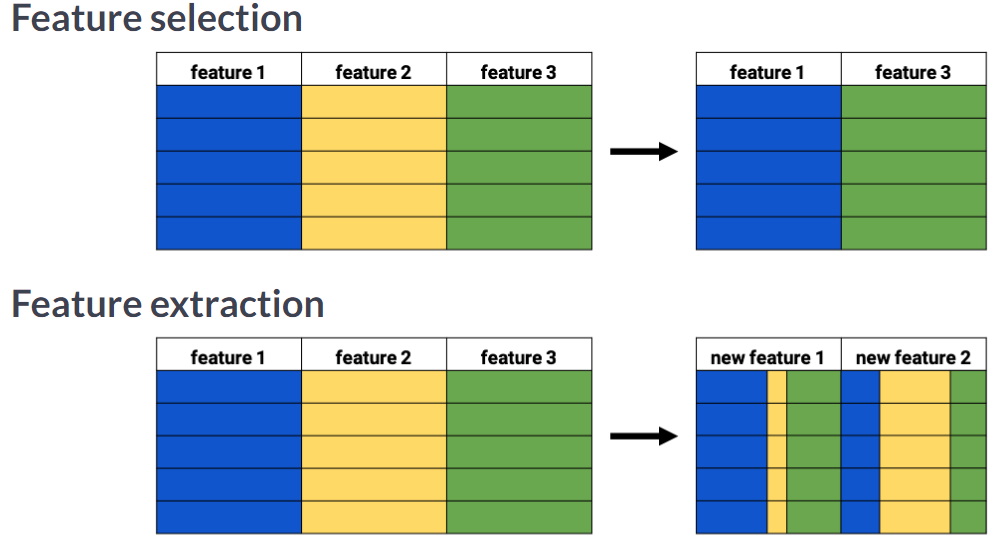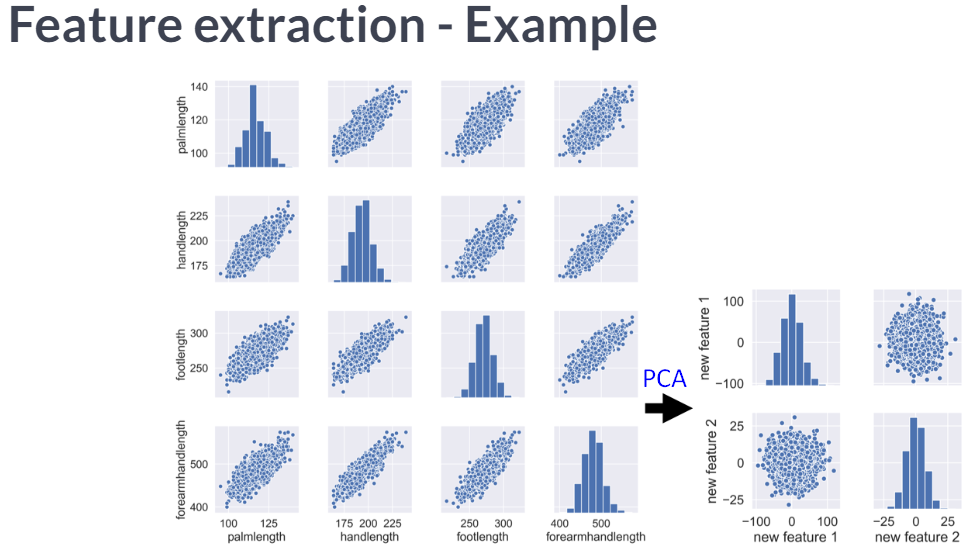## Visually detecting redundant features

Data visualization is a crucial step in any data exploration. Let’s use Seaborn to explore some samples of the US Army ANSUR body measurement dataset.

Two data samples have been pre-loaded as `ansur_df_1` and `ansur_df_2`.

Seaborn has been imported as `sns`.

``````# Create a pairplot and color the points using the 'Gender' feature
sns.pairplot(ansur_df_1, hue='Gender', diag_kind='hist')
plt.show()
``````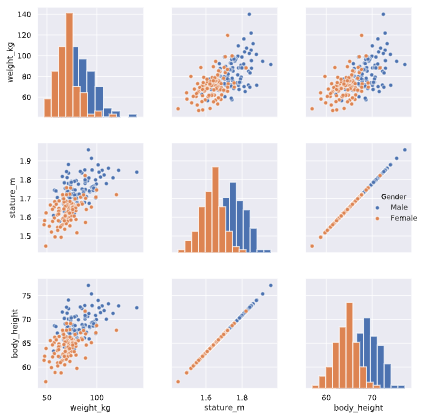Two features are basically duplicates, remove one of them from the dataset.

``````# Remove one of the redundant features
reduced_df = ansur_df_1.drop('stature_m', axis=1)

# Create a pairplot and color the points using the 'Gender' feature
sns.pairplot(reduced_df, hue='Gender')
# Show the plot
plt.show()
``````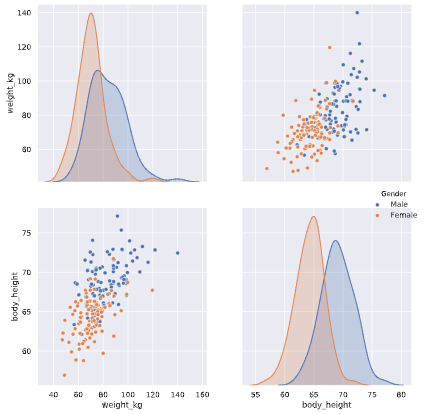``````# Create a pairplot and color the points using the 'Gender' feature
sns.pairplot(ansur_df_2, hue='Gender', diag_kind='hist')
plt.show()
``````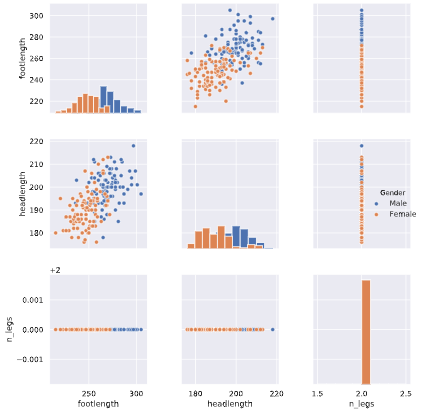One feature has no variance, remove it from the dataset.

``````# Remove the redundant feature
reduced_df = ansur_df_2.drop('n_legs', axis=1)

# Create a pairplot and color the points using the 'Gender' feature
sns.pairplot(reduced_df, hue='Gender', diag_kind='hist')
# Show the plot
plt.show()
``````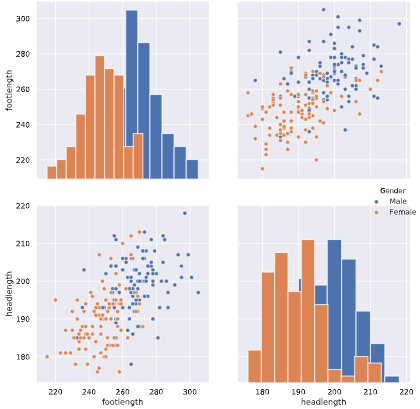The body height (inches) and stature (meters) hold the same information in a different unit + all the individuals in the second sample have two legs.

What advantage does feature selection have over feature extraction?

Answer: The selected features remain unchanged, and are therefore easy to interpret.

Extracted features can be quite hard to interpret.

#python #data #dimensionality-reduction1596851760

## Dimensionality Reduction in Machine Learning

why We use Dimensionality Reduction Technique?

Human Being are Can’t visualize the High Dimensional data so we want to reduce in to low dimension.In real world data analysis tasks we analyze complex data i.e. multi dimensional data. We plot the data and find various patterns in it or use it to train some machine learning models. One way to think about dimensions is that suppose you have an data point _x _, if we consider this data point as a physical object then dimensions are merely a basis of view, like where is the data located when it is observed from horizontal axis or vertical axis.

As the dimensions of data increases, the difficulty to visualize it and perform computations on it also increases. So, how to reduce the dimensions of a data-

* Remove the redundant dimensions

* Only keep the most important dimensions

what are the Techniques in the Dimensionality Reduction in Machine Learning?

In this article We use the Fundamental Techniques Like PCA and t-SNE .

_Principal Component Analysis(PCA): _In Machine Learning PCA is the Unsupervised Learning Technique .

First try to understand some terms

_Variance : _It is a measure of the variability or it simply measures how spread the data set is. Mathematically, it is the average squared deviation from the mean score. We use the following formula to compute variance var(x).

#data-science #towards-data-science #machine-learning #data-visualization #dimensionality-reduction